# Planetary Motion¶

### Example - Astrophysics¶

Last edited: March 16th 2018

In the modules under ordinary differential equations we have looked at different methods for solving ordinary differential equations (ODE's), and briefly discussed the accuracy of these methods. In this example we will apply the explicit and implicit Euler methods, and the fourth order Runge-Kutta method to calculate the trajectory of the Earth around the Sun. This will show how the accuracy of the methods affect the calculated trajectory.

### Equations of Motion¶

We will simplify the problem by neglecting the gravitational force on the Earth from all other planets, hence only considering the force between the Earth and the Sun. Newton's second law then yields the following equations of motion

$$m\vec{a} = m\ddot{\vec{r}}= -\frac{GMm}{r^3}\vec{r},$$

where $m$ is the mass of the Earth, $M$ is the mass of the Sun, $r=|\vec{r}|$ is the distance between the Sun and Earth, and $G$ is the gravitational constant. We will try to write this in dimensionless form, using the Aphelion seperation $R$ between the Earth and Sun as a unit, and the period $T$ as time unit. Hence we define

$$\tau \equiv\frac{t}{T},\,\rho \equiv \frac{r}{R},\,X \equiv\frac{x}{R}\,\mathrm{and}\,Y\equiv\frac{y}{R}.$$

Inserting this into the equation of motion, dividing away the common factor $m$, we get

$$\frac{R}{T^2}\frac{d^2\vec{\rho}}{d\tau^2} = -\frac{GM}{R^2\rho^3}\vec{\rho}.$$

Let's see if we have done this right by checking that the remaining constant is dimensionless,

$$\left[\frac{GMT^2}{R^3}\right] = \frac{\mathrm{N(m/kg)^2\cdot kg \cdot s^2}}{m^3} = 1.$$

We define the constant $$C \equiv \frac{GMT^2}{R^3},$$

and get the dimensionless equations of motion

$$\frac{d^2\vec{\rho}}{d\tau^2} = -C \frac{\vec{\rho}}{\rho^3}.$$

### Numerical Implementation¶

Since the angular momentum of the system is conserved in this problem, we will consider the motion of the Earth in a plane, where the Sun is stationary at the origin. Hence we have that $\vec{\rho} = X\hat{x} + Y\hat{y}$, and we want to rewrite the above equation as four first order ODEs in order to use the mentioned numerical methods. We get

\begin{align} \frac{dX}{d\tau} &= U,\qquad \frac{dU}{d\tau} = -C\frac{X}{\rho^3},\\ \frac{dY}{d\tau} &= V,\qquad \frac{dV}{d\tau} = -C\frac{Y}{\rho^3}, \end{align}

where $U$ and $V$ are the dimensionless velocities in the $x$- and $y$-direction, and $\rho=\sqrt{X^2+Y^2}$.

We'll use initial conditions

\begin{align} x_0&=R \,&\Rightarrow\, &&&X_0=1,\\ v_0 &= 30.29 \mathrm{ km/s} \,&\Rightarrow\, &&&V_0 = \frac{u T}{R} = 29.29\cdot 10^3 \mathrm{m/s} \frac{365.25 \cdot 24 \cdot 3600 \mathrm{s}}{152.10\cdot 10^9 \mathrm{m}} \approx 6.33, \end{align}

and $Y_0 = 0$, $U_0 = 0$.

We are now ready to start implementing the different methods. We will use $r$ instead of $\rho$ below. First we import the needed libraries and define the global constants.

In :
%matplotlib inline
import numpy as np
import matplotlib.pyplot as plt
from matplotlib import rc

#Set common figure parameters:
newparams = {
'figure.figsize': (16, 5), 'axes.grid': True,
'lines.linewidth': 1.5, 'font.size': 19, 'lines.markersize' : 10,
'mathtext.fontset': 'stix', 'font.family': 'STIXGeneral'}
plt.rcParams.update(newparams)

T = 365.25*24*3600  # Orbital period [s]
R = 152.10e9        # Aphelion distance [m]
G = 6.673e-11       # Gravitational constant [N (m/kg)^2]
M = 1.9891e30       # Solar mass [kg]
m = 5.9726e24       # Earth mass [kg]

C = (G*M*T**2)/(R**3)
#print(C)

# Initial conditions
X0 = 1
U0 = 0
Y0 = 0
V0 = 29.29e3*T/R

t_max = 1    # t_max=1 Corresponds to one single complete orbit
dt = 0.0001
N = int(t_max/dt)  # Number of time steps


Let us now use the different numerical methods to calculate the trajectory. In order to see how good the methods are, we will consider the following:

• The energy should be conserved, and hence the orbit should be closed.
• We know the perihelion seperation (the minimum distance to the Sun), $\rho_\mathrm{perihelion} = 0.967$, and can check how close the trajectory is to this point.

Let's begin!

### Explicit Euler Method¶

We first a function which returns the RHS of the four ODEs given above, before implementing the explicit Euler method.

In :
def RHS(x, y, u, v):
""" Returns the time derivatives of X, Y, U and V. Also returns the total energy E of the system."""

r = np.sqrt(x**2 + y**2)
dxdt = u
dydt = v
dudt = -C*x/r**3
dvdt = -C*y/r**3

E = 0.5*(u**2+v**2)-C/r

return (dxdt, dydt, dudt, dvdt, E)

X = np.zeros(N)
Y = np.zeros(N)
U = np.zeros(N)
V = np.zeros(N)
E = np.zeros(N-1)  # Total energy/mass

X = X0
V = V0

for n in range(N-1):
(dX, dY, dU, dV, E[n]) = RHS(X[n], Y[n], U[n], V[n])
X[n+1] = X[n] + dt*dX
Y[n+1] = Y[n] + dt*dY
U[n+1] = U[n] + dt*dU
V[n+1] = V[n] + dt*dV

# If t_max was set to exactly one period, it will be interesting
# to investigate whether or not the orbit is closed (planet returns
# to its starting position)
if(t_max==1):
# Find offset
print("\nSmall offset indicates closed orbit")
print("Offset in 'x': %0.3e - %0.7e = %0.7e" % (X, X[-1], X[-1]-X))
print("Offset in 'y': %0.3e - %0.7e = %0.7e" % (Y, Y[-1], Y[-1]-Y))
print("Total offset: %0.3e" % np.sqrt((X-X[-1])**2+(Y-Y[-1])**2))

# Find perihelion seperation:
r_perihelion = abs(min(Y))
print("\nThe perihelion seperation is %0.3f, compared to 0.967." % r_perihelion)

plt.figure()
plt.plot(X, Y, 'g', , , 'ro')
plt.title("Explicit Euler")
plt.xlabel(r"$x$")
plt.ylabel(r"$y$")
plt.grid()

plt.figure()
plt.plot(E)
plt.title('Energy per unit mass')
plt.ylabel("Energy")
plt.xlabel(r"$n$")
plt.grid();

Small offset indicates closed orbit
Offset in 'x': 1.000e+00 - 1.0073783e+00 = 7.3783114e-03
Offset in 'y': 0.000e+00 - -3.4487638e-02 = -3.4487638e-02
Total offset: 3.527e-02

The perihelion seperation is 0.990, compared to 0.967.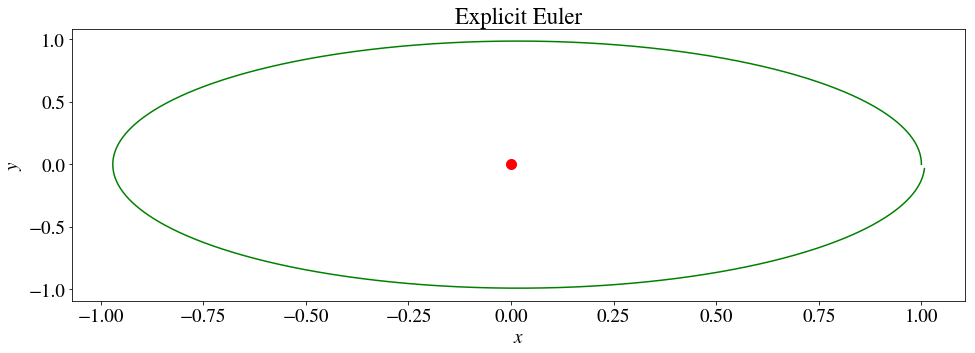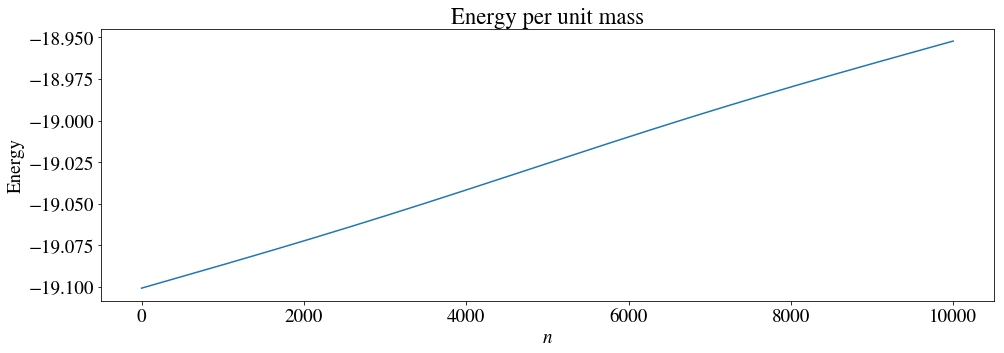### Implicit Euler Method¶

When using the Implicit Euler Method, we evaluate the RHSs of the ODEs at the new timestep. We hence get the following equations \begin{align} X_{n+1} &= X_{n} + \Delta t U_{n+1},\\ U_{n+1} &= U_n - \Delta t C\frac{X_{n+1}}{\rho_{n+1}^3},\\ Y_{n+1} &= Y_{n} + \Delta t V_{n+1},\\ V_{n+1} &= V_n - \Delta t C\frac{Y_{n+1}}{\rho_{n+1}^3}. \end{align} We will use the root solver,

scipy.optimize.root,



to find the values at the new time step in the equations above.

In :
from scipy.optimize import root

def func(X, X0):
""" Function returning the difference between the RHS and LHS of the four first order ODEs above.

X:     vector of new coordinates
X0:    vector of old coordinates

"""

X_ = X
Y_ = X
U_ = X
V_ = X

X0_ = X0
Y0_ = X0
U0_ = X0
V0_ = X0

r = np.sqrt(X_**2 + Y_**2)

return [X_ - (X0_ + dt*U_),
Y_ - (Y0_ + dt*V_),
U_ - (U0_ - dt*C*X_/r**3),
V_ - (V0_ - dt*C*Y_/r**3)]

X = np.zeros(N)
Y = np.zeros(N)
U = np.zeros(N)
V = np.zeros(N)

X = X0
V = V0

for n in range(N-1):
X0_ = np.array((X[n], Y[n], U[n], V[n]))
sol = root(func, x0=X0_, args=X0_, jac=False)

X[n+1] = sol.x
Y[n+1] = sol.x
U[n+1] = sol.x
V[n+1] = sol.x

# If t_max was set to exactly one period, it will be interesting
# to investigate whether or not the orbit is closed (planet returns
# to its starting position)
if(t_max==1):
# Find offset
print("\nSmall offset indicates closed orbit")
print("Offset in 'x': %0.3e - %0.7e = %0.7e" % (X, X[-1], X[-1]-X))
print("Offset in 'y': %0.3e - %0.7e = %0.7e" % (Y, Y[-1], Y[-1]-Y))
print("Total offset: %0.3e" % np.sqrt((X-X[-1])**2+(Y-Y[-1])**2))

# Find perihelion seperation:
r_perihelion = abs(min(Y))
print("\nThe perihelion seperation is %0.3f, compared to 0.967." % r_perihelion)

plt.figure()
plt.plot(X, Y, 'g', , , 'ro')
plt.title("Implicit Euler")
plt.xlabel(r"$x$")
plt.ylabel(r"$y$")
plt.grid()

# Calculate energy
r = np.sqrt(X**2 + Y**2)
E = 0.5*(U**2+V**2)-C/r

plt.figure()
plt.plot(E)
plt.title('Energy per unit mass')
plt.ylabel("Energy")
plt.xlabel(r"$n$")
plt.grid();

Small offset indicates closed orbit
Offset in 'x': 1.000e+00 - 9.9124581e-01 = -8.7541899e-03
Offset in 'y': 0.000e+00 - 3.7544951e-02 = 3.7544951e-02
Total offset: 3.855e-02

The perihelion seperation is 0.976, compared to 0.967.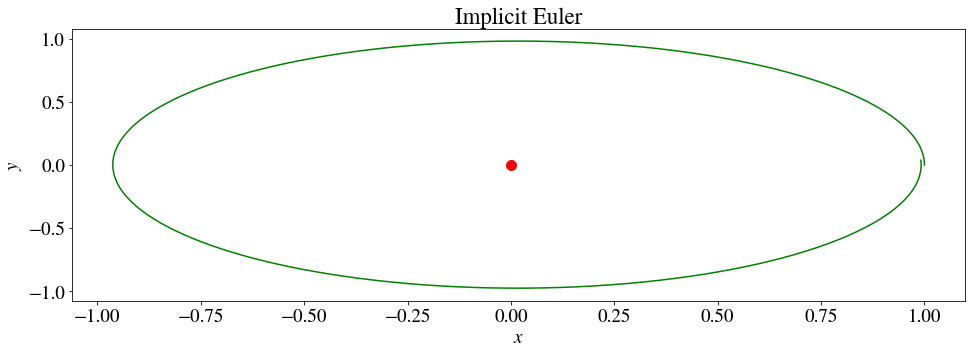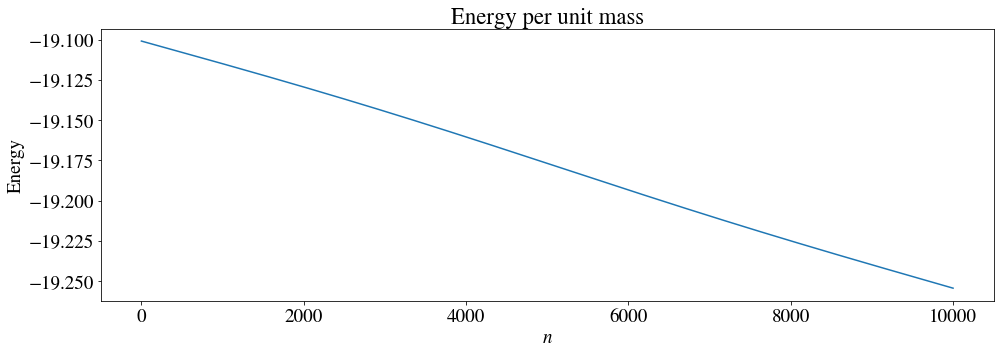### Fourth Order Runge-Kutta Method¶

We will in the following solve the system one last time, utilzing the Fourth Order Runge-Kutta Method (RKM4). Since the RKM4 makes use of test-points, we'll separate the four equations into separate functions.

In :
def F(X_, Y_, U_, V_): # dX/dtau
return U_

def G(X_, Y_, U_, V_): # dY/dtau
return V_

def H(X_, Y_, U_, V_): # dU/dtau
return -C * (  X_/( (np.sqrt(X_**2 + Y_**2) )**3)  )

def I(X_, Y_, U_, V_): # dV/dtau
return -C * (  Y_/( (np.sqrt(X_**2 + Y_**2) )**3)  )

X_4RK = np.zeros(N)
Y_4RK = np.zeros(N)
U_4RK = np.zeros(N)
V_4RK = np.zeros(N)
E_4RK = np.zeros(N-1)  # Total energy/mass

X_4RK = X0
V_4RK = V0

for n in range(N-1):

k_x1 = dt * F( X_4RK[n], Y_4RK[n], U_4RK[n], V_4RK[n] )
k_y1 = dt * G( X_4RK[n], Y_4RK[n], U_4RK[n], V_4RK[n] )
k_u1 = dt * H( X_4RK[n], Y_4RK[n], U_4RK[n], V_4RK[n] )
k_v1 = dt * I( X_4RK[n], Y_4RK[n], U_4RK[n], V_4RK[n] )

k_x2 = dt * F( X_4RK[n] + k_x1/2, Y_4RK[n] + k_y1/2, U_4RK[n] + k_u1/2, V_4RK[n] + k_v1/2 )
k_y2 = dt * G( X_4RK[n] + k_x1/2, Y_4RK[n] + k_y1/2, U_4RK[n] + k_u1/2, V_4RK[n] + k_v1/2 )
k_u2 = dt * H( X_4RK[n] + k_x1/2, Y_4RK[n] + k_y1/2, U_4RK[n] + k_u1/2, V_4RK[n] + k_v1/2 )
k_v2 = dt * I( X_4RK[n] + k_x1/2, Y_4RK[n] + k_y1/2, U_4RK[n] + k_u1/2, V_4RK[n] + k_v1/2 )

k_x3 = dt * F( X_4RK[n] + k_x2/2, Y_4RK[n] + k_y2/2, U_4RK[n] + k_u2/2, V_4RK[n] + k_v2/2 )
k_y3 = dt * G( X_4RK[n] + k_x2/2, Y_4RK[n] + k_y2/2, U_4RK[n] + k_u2/2, V_4RK[n] + k_v2/2 )
k_u3 = dt * H( X_4RK[n] + k_x2/2, Y_4RK[n] + k_y2/2, U_4RK[n] + k_u2/2, V_4RK[n] + k_v2/2 )
k_v3 = dt * I( X_4RK[n] + k_x2/2, Y_4RK[n] + k_y2/2, U_4RK[n] + k_u2/2, V_4RK[n] + k_v2/2 )

k_x4 = dt * F( X_4RK[n] + k_x3, Y_4RK[n] + k_y3, U_4RK[n] + k_u3, V_4RK[n] + k_v3 )
k_y4 = dt * G( X_4RK[n] + k_x3, Y_4RK[n] + k_y3, U_4RK[n] + k_u3, V_4RK[n] + k_v3 )
k_u4 = dt * H( X_4RK[n] + k_x3, Y_4RK[n] + k_y3, U_4RK[n] + k_u3, V_4RK[n] + k_v3 )
k_v4 = dt * I( X_4RK[n] + k_x3, Y_4RK[n] + k_y3, U_4RK[n] + k_u3, V_4RK[n] + k_v3 )

X_4RK[n+1] = X_4RK[n] + k_x1/6 + k_x2/3 + k_x3/3 + k_x4/6
Y_4RK[n+1] = Y_4RK[n] + k_y1/6 + k_y2/3 + k_y3/3 + k_y4/6
U_4RK[n+1] = U_4RK[n] + k_u1/6 + k_u2/3 + k_u3/3 + k_u4/6
V_4RK[n+1] = V_4RK[n] + k_v1/6 + k_v2/3 + k_v3/3 + k_v4/6

E_4RK[n] = 0.5*(U_4RK[n+1]**2+V_4RK[n+1]**2)-C/np.sqrt(X_4RK[n+1]**2 + Y_4RK[n+1]**2)

# If t_max was set to exactly one period, it will be interesting
# to investigate whether or not the orbit is closed (planet returns
# to its starting position)
if(t_max==1):
# Find offset
print("\nSmall offset indicates closed orbit")
print("Offset in 'x': %0.3e - %0.7e = %0.7e" % (X_4RK, X_4RK[-1], X_4RK[N-1]-X_4RK))
print("Offset in 'y': %0.3e - %0.7e = %0.7e" % (Y_4RK, Y_4RK[-1], Y_4RK[N-1]-Y_4RK))
print("Total offset: %0.3e" % np.sqrt((X_4RK-X_4RK[-1])**2+(Y_4RK-Y_4RK[-1])**2))

# Find perihelion seperation:
r_perihelion = abs(min(Y_4RK))
print("\nThe parahelion seperation is %0.3f, compared to 0.967." % r_perihelion)

plt.figure()
plt.title('4th order Runge-Kutta')
plt.plot(X_4RK, Y_4RK, 'g', , , 'ro')
plt.xlabel(r"$x$")
plt.ylabel(r"$y$")
plt.grid()

plt.figure()
plt.title('Energy per unit mass')
plt.plot(E_4RK)
plt.ylabel("Energy")
plt.xlabel(r"$n$")
plt.grid()

Small offset indicates closed orbit
Offset in 'x': 1.000e+00 - 9.9999892e-01 = -1.0753348e-06
Offset in 'y': 0.000e+00 - 1.4540584e-03 = 1.4540584e-03
Total offset: 1.454e-03

The parahelion seperation is 0.983, compared to 0.967.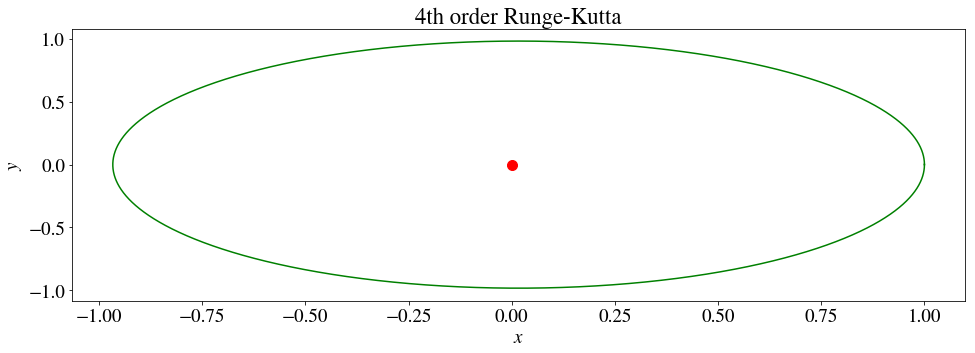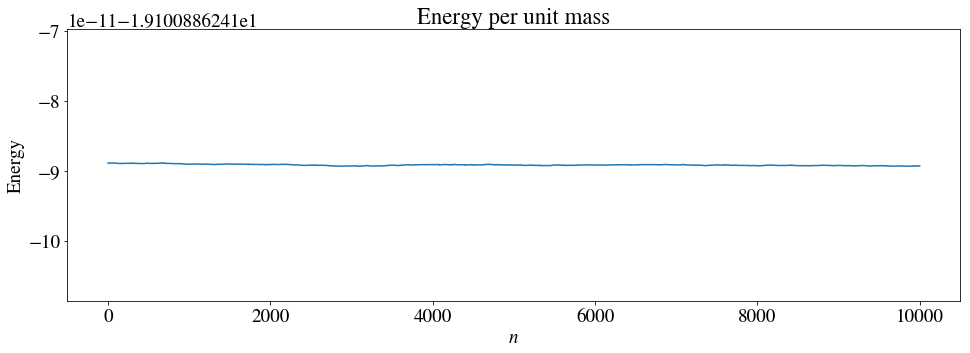From the above results we see that the Runge-Kutta method is in fact the most accurate one: while the plot of energy/mass for the two first methods show large deviations, the energy/mass is close to constant for the RKM4. This leads to a nearly to closed orbit. This should not be surprising as the RKM4 is implemented as a 4th-order method, and hence should be superior to the explicit and implicit Euler methods, which are 1st-order methods, in terms of accuracy.